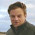## Tuesday, June 25, 2013

### Performance of Scala Iterators

Objective
The Scala programming language provides software developers with several options to iterate through the elements of a collection:

• for,while loops and foreach ( x => f(x)) higher order function.
• map[Y] { f: X => Y) : Collection[Y] that creates a new collection by applying a function f to each element of the collection
• foldLeft[Y] (y : Y)(f : (Y,X)=>Y) : Y) (resp. foldRight[Y] (y : Y)(f : (X,Y)=>Y) : Y) that applies a binary operator to each element of this collection starting left to right (resp. right to left)

This post attempts to quantify the overhead of the most commonly used iterative methods in Scala and demonstrate the effectiveness of the higher order methods map and foldLeft

Scala loops for summation
The test runs are executed on a 'plain vanilla' dual core i3 2.1 Ghz running Linux CentOs 6.0. The first test consists of comparing compare the performance of the different options to traverse an array of Float with size varies from 2,000,000 to 40,000,000 elements then apply an operation += z to each of its members. The options are
  foreach   (line 6)
for loop  (line 9)
while loop (lines 14 - 16)
foldLeft   (line 19)


  1 2 3 4 5 6 7 8 9 10 11 12 13 14 15 16 17 18 19 val rGen = new scala.util.Random(System.currentTimeMillis) var data = Array.fill(size)(rGen.nextFloat) var sum = 0.0 // Higher order method data.foreach(sum += _) // for loop for( x <- data) sum += x // while loop var k = 0 val len = data.size while( k < len) { sum += data(k) k += 1 } // fold sum = data.foldLeft(0.0)((x, z) => x + z) 

The test is repeated 25 times in order to reduce variance and noise generated by the garbage collector. The first 5 iterations are discarded to avoid the overhead of the initialization of the JVM. The mean value of the execution time for each method is computed for different size of an array from 2,000,000 to 40,000,000 floating point values (type Float). The results of the test are plotted in the graph below. The unit of time on the Y-coordinate is milliseconds.
The for, while and foreach expression have very similar performance.
The foldLeft is significantly faster (ratio 1:6)

Data transformation
The second test consists of comparing the performance of
• foreach: "fills-up" iteratively a mutable array of type ArrayBuffer (line 3)
• foreach: creates and updates a copy of the original array (immutable approach)(lines 7 & 8)
• map: transform the original array into an array of square values (line 11)

  1 2 3 4 5 6 7 8 9 10 11  // foreach with mutable array buffer val newData = new mutable.ArrayBuffer[Float] data.foreach( (x: Float) => newData.append(x *x)) val result = newData.toArray // foreach with update of immmutable array val pData = Array.fill(sz)(0.0) data.foreach( pData.update(i, _) ) // map val nData = data.map((x:Float) => x*x) 

Let's run the same test (with the same setup defined in the previous section).

The test shows that the method dedicated to convert an array to other array by applying a natural transformation, map is by far the most efficient.
The methods dedicated to a specific task such as foldLeft for summation and map for data transformation are far more effective that the "plain vanilla" loop constructors. The tests are conducted with Scala 2.10.2

Important Notes:
The syntax or construct for has a very different meaning in Scsla as in C or Java. It is actually a wrapper or syntactic sugar layer around the monadic chain of flatMap and map transformation as follows

  for (
a <- f(x)  // flatMap
b <- g(a)  // flatMap
c <- h(b)  // map
) yield { }

Therefore, it should be avoided as an iterative counter and reserved to be used as for-comprehensive construct.

A more elaborate and time consuming benchmark would consist of running multiple tests using several boolean (< !=..) and numeric (+, *, x => sin(x) ..) operators and computes the normalize mean and variance.

References
Scala for the Impatient - C. Horstman - Addison-Wesley 2012
github.com/prnicolas

1.your "for-loop" is a very bad example, it is no normal C loop. If you realy wanted to compare C loops against scala iterators you would have to use a while.

like this:
var i = 0
while(i < data.length()){ ...; i += 1}

2.First of all, running a test only 10 times is not enough to guarantee that JIT has warmed up. But I'm going to let it pass. It's an understandable mistake.

In the first test, the foreach loop does absolutely nothing. data.foreach( x => x + z) == () and nothing is modified, map creates a copy of the array, and for modifies the array in place. And finally, in the second test, foreach again does nothing, map does something barely sensible (creates a new list in a very contrived way) and foldLeft... returns the last element of the list.

Of course the results are gonna vary, each of the tested methods in both cases does absolutely different things. It's not even comparing apples to oranges, it's comparing Apple Inc. to oranges.

3.The purpose of the article was to quantify
- Overhead of the for loop in Scala (while loop as to be used instead a for comprehensive)
- Cost of some Scala iterators (without any operation)
I take it the objective of the post is unclear...

4.here are results for Scala 2.11:
https://github.com/maciej/iterators-benchmark

5.I really baffles me that while performs so badly. How could .sum possibly be faster, except if it uses parallellism, which is shouldn't by default.

By the way, did you compile the program using the scalac -optimize? You should have.